# Class 6 Maths NCERT Solutions for Chapter 6 Integers Ex – 6.2

## Integers

Question 1.
Using the number line write the integer which is:

(a) 3 more than 5
(b) 5 more than -5
(c) 6 less than 2
(d) 3 less than -2

Solution:
(a)
We want to obtain integer 3 more than 5. So, we start from 5 and proceed 3 units to the right to obtain 8 as shown in the figure.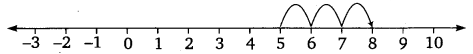Hence, 3 more than 5 is 8.
(b) We want to obtain integer 5 more than -5. So, we start from -5 and proceed 5 units to the right to obtain 0 as shown in the figure.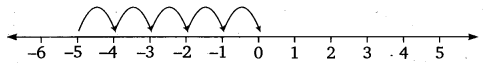Hence, 5 more than -5 is 0.
(c) We want to obtain an integer 6 less than 2. So, we start from 2 and proceed 6 units to the left of it to obtain -4 as shown in the figure.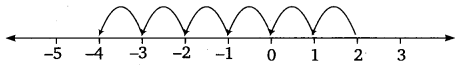Hence, 6 less than 2 is -4.
(d) We want to obtain an integer 3 less than -2. So, we start from -2 and proceed 3 units to the left of it to obtain -5 as shown in the figure.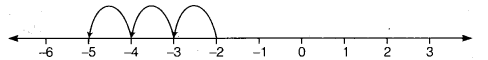Hence, 3 less than -2 is -5.

Question 2.
Use number line and add the following integers:

(a) 9 + (-6)
(b) 5 + (-11)
(c) (-1) + (-7)
(d) (-5) + 10
(e) (-1) + (-2) + (-3)
(f) (-2) + 8 + (-4)

Solution:
(a)
First we move to the right of 0 by 9 steps reaching 9. Then we move 6 steps to the left of 9 reaching 3 as shown in the figure.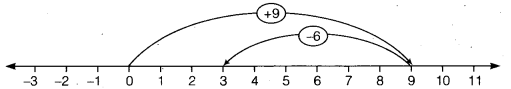Thus, 9 + (-6) = 3.
(b) First we move to the right of 0 by 5 steps reaching 5. Then, we move 11 steps to the left of 5 reaching -6 as shown in the figure.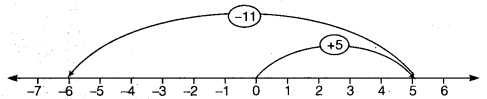Thus, 5 + (-11) = -6.
(c) First we move to the left of 0 by 1 step reaching -1. Then we move 7 steps to* the left of -1 reaching -8 as shown in the figure.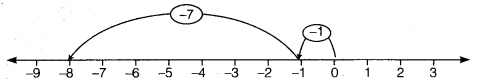Thus, (-1) + (-7) = -8.
(d) First we move to the left of 0 by 5 steps reaching -5. Then we move 10 steps to the right of -5 reaching 5 as shown in the figure.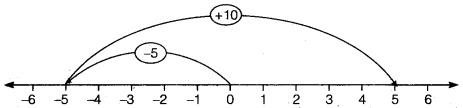Thus, (-5) + 10 = 5.
(e) First we move to the left of 0 by 1 step reaching -1. Then we move 2 steps to the left of -1 reaching -3. Again, we move 3 steps to the left of -3 reaching -6 as shown in the figure.Thus, (-1) + (-2) + (-3) = -6.
(f) First we move to the left of 0 by 2 steps reaching -2. Then we move 8 steps to the right of -2 reaching 6. Again, we move 4 steps to the left of 6 reaching -2 as shown in the figure.
Thus, (-2) + 8 + (-4) = 2.Question 3.

(a) 11 + (-7)
(b) (-13) + (+18)
(c) (-10) + (+19)
(d) (-250) + (+150)
(e) (-380)+ (-270)
(f) (-217) + (-100)

Solution:
(a)
11 + (-7) = 4 + 7 + (-7)
= 4 + 0 = 4
(b) (-13) + (+18) = (-13) + (+13) + (+ 5)
= 0 + (+5) = 5
(c) (-10) + (+19) = (-10) + (+10) + (+9)
= 0 + (+9) = +9
(d) (-250) + (+150) = (-100) + (-150) + (+150)
= (-100) + 0 = -100
(e) (-380) + (-270) = -650
(f) (-217) + (-100) = -317

Question 4.
Find the sum of:

(a) 137 and -354
(b) -52 and 52
(c) -312, 39 and 192
(d) -50, – 200 and 300

Solution:
(a)
(+137) + (-354) = (+137) + (-137) + (-217)
= 0 + (-217) =-217
(b) (-52) + (+52) = 0
(c) (-312) + (+39) + (+192) = (-312) + (+231)
= (-81) + (-231) + (+231)
= (-81) + 0 = -81
(d) (-50) + (-200) + (+300) = (-250) + (+300)
= (-250) + (+250) + (+50)
= 0 + (+50) = +50

Question 5.
Find the sum:

(a) (-7) + (-9) + 4 + 16
(b) (37) + (-2) + (-65) + (-8)

Solution:
(a) (-7) + (-9) + 4 + 16 = (-16) + 4 + 16
= (-16) + (+16) + 4 = 0 + 4 = 4
(b) (37) + (-2) + (-65) + (-8) = 37 + (-75)
= 37+ (-37)+ (-38)
= 0 + (-38) = -38

### Take Your IIT JEE Coaching to Next Level with Examtube

• Mentoring & Teaching by IITians
• Regular Testing & Analysis
• Preparation for Various Engineering Entrance Exams
• Support for School/Board Exams